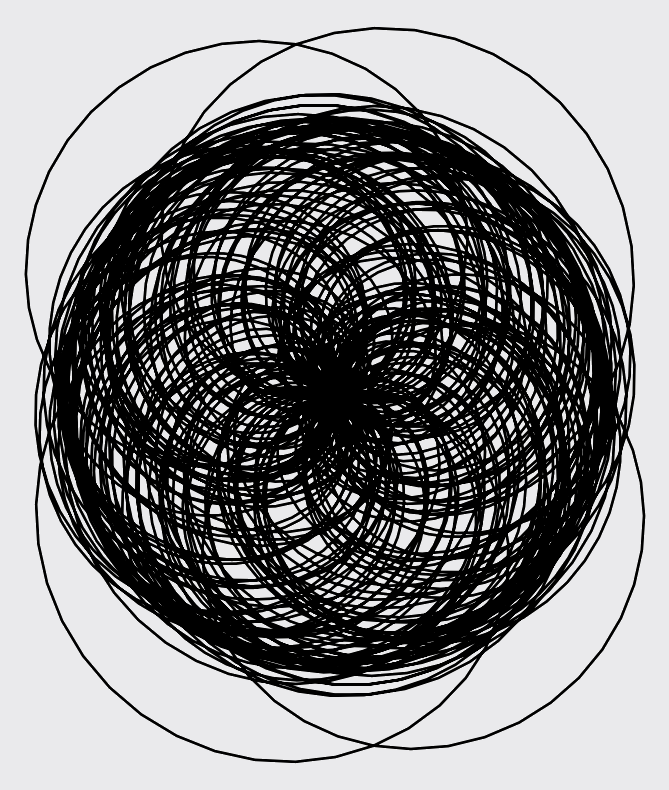# Dirichlet Characters

In mathematics, a character is a well-behaved function from a group to a field. In number theory, the most common characters are Dirichlet characters, which are functions from the integers to the unit circle.

More precisely, a Dirichlet character $$\chi$$ is a multiplicative function $$\chi : (\mathbb{Z}/N\mathbb{Z})^\times \longrightarrow \mathbb{C}.$$

Every Dirichlet character has a label consisting of two integers, $$N$$ and $$a$$. The character $$\chi(N, a)$$ (sometimes written $$\chi_N(a, \cdot)$$) is defined modulo $$N$$ and has index $$a$$.

The idea of plotting a Dirichlet character is a bit odd, since the "image" consists of several points on the unit circle. These plots would highly resemble various approximations of the circle --- mostly uninteresting. But a character can be interpolated to a function $$\chi : \mathbb{R} \longrightarrow \mathbb{C}$$ through a Gauss sum $$\tau(\chi) := \sum_{n \bmod N} \chi(n) \exp(2 \pi i n / N).$$ One can prove the identity $$\chi(x) = \frac{\chi(-1) \tau(\overline{\chi})}{N} \sum_{n \bmod N} \overline{\chi}(n) \exp(2 \pi i n x / N),$$ and note that this function makes sense for all $$x \in \mathbb{R}$$. On integers, it agrees with the typical Dirichlet character.

Now it is possible to plot many characters. These are the characters mod $$22$$, excluding the trivial character.

Some characters look very simple.

Others look more complicated.

Others look downright busy.

But as one continues to make and study these images, patterns emerge. A curtain hides an underlying image --- subsequent characters shed light, but the image remains obscured.

Or there may be a feeling like someone is watching you.

Even the busy character above seems to be one part in a sequence. The next term might look like the following.For characters with larger moduli, the images take on a different facet of beauty.

The clustering around the center and around an apparent circle are not coincidental. At integer inputs $n$, $\chi(n)$ will either be $0$ or lie on the unit circle. In many images, the tension comes from the the apparent randomness and the need to return to the circle.

Prints of high-res versions of these images are available. Contact me at mixedmathart@lowryduda.com for more.